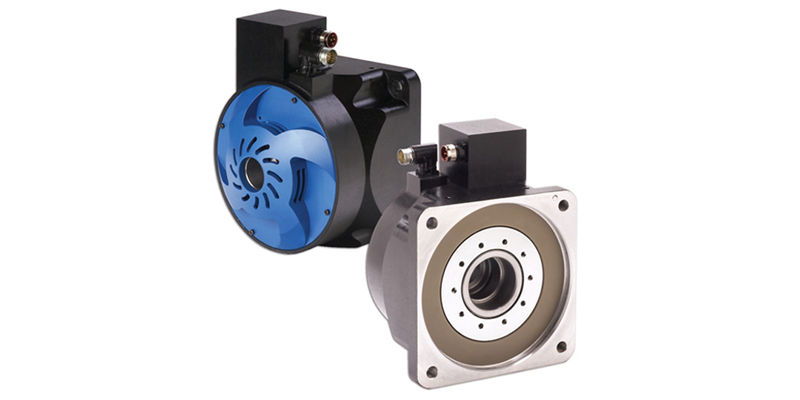blog | 超越性能：直驱系统的成本节约 |
|### 降低零件成本

= 电机成本 – [(减速机) + (同步带) + (皮带轮) + (丝杠) + (轴封) + (轴承)]

### 降低劳动力成本

= (人工费率) + (小时数) + (设备数)

### 降低维护成本

Maintenance Costs
= [(减速机) + (同步带) + (皮带轮) + (丝杠) + (轴封) + (轴承)] + (人工费率) + (小时数)

### 缩短停机时间，增加收入

= (停机周期) + (零件生产损失+ 单零件成本)

### 性能更好，产量更高

= ((lx – ly)/T) + (单零件)

### 综合所有优势

• 零件成本节约 = 电机成本–[（齿轮）+（正时皮带）+（皮带轮）+（丝杠）+（密封件）+（轴承）]
• 安装劳动力节约 =（人工费率）*（工时）*（设备数量）
• 维护成本 = [（齿轮）+（正时皮带）+（皮带轮）+（丝杠）+（密封件）+（轴承）]+（人工费率 * 工时）
• 停机成本 = 停机持续时间*（单位产量损失 * 单位成本）
• 总收入 =（（Ix–Iy）/T）* 单位成本